# ISEE Upper Level Quantitative : How to find an angle

## Example Questions

2 Next →

### Example Question #11 : Isee Upper Level (Grades 9 12) Quantitative Reasoning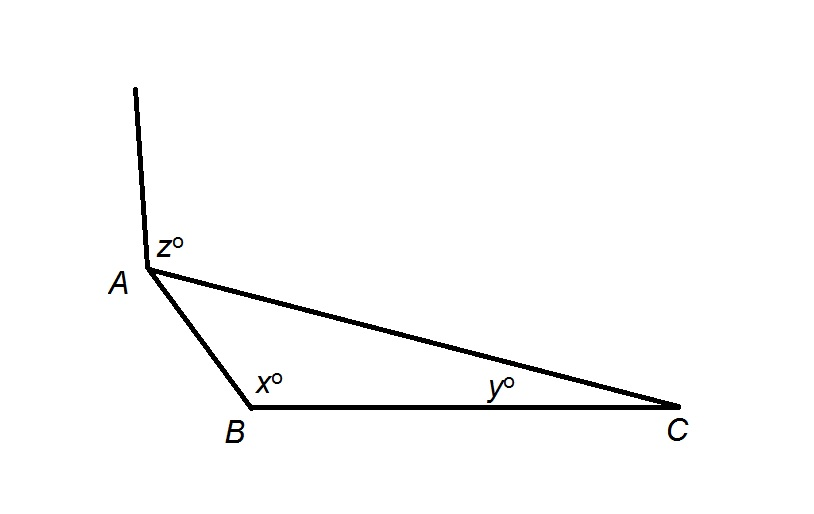Refer to the above figure. Which is the greater quantity?

(a)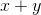(b)(b) is the greater quantity

(a) is the greater quantity

(a) and (b) are equal

It is impossible to determine which is greater from the information given

(a) is the greater quantity

Explanation:

Extend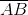as seen in the figure below: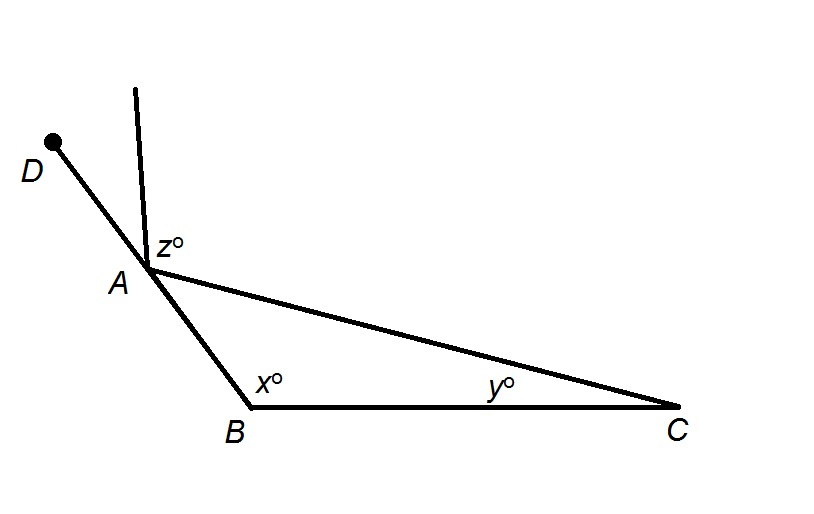The measure of an exterior angle of a triangle is equal to the sum of the measures of its remote interior angles; specifically,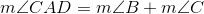,

and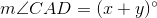However,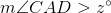, so, by substitution,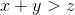### Example Question #12 : Geometry

Given: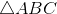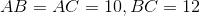. Which is the greater quantity?

(a)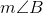(b)(a) and (b) are equal

(b) is the greater quantity

It is impossible to determine which is greater from the information given

(a) is the greater quantity

(b) is the greater quantity

Explanation:

Below is the referenced triangle along with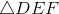, an equilateral triangle with sides of length 10: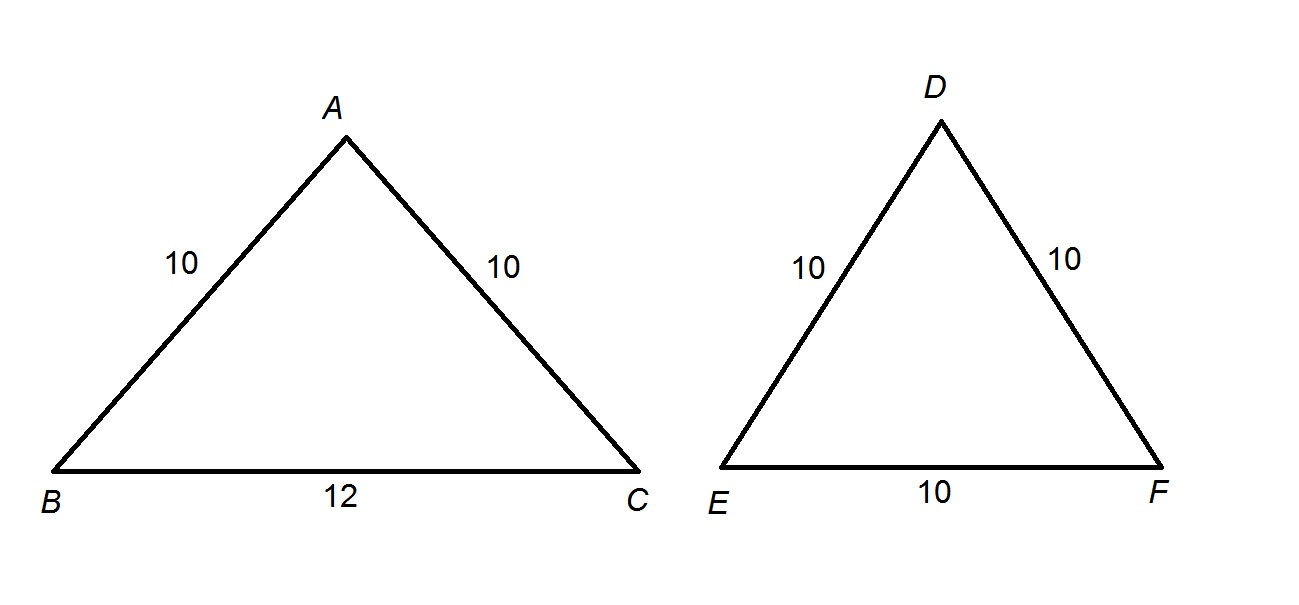As an angle of an equilateral triangle,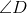has measure. Applying the Side-Side-Side Inequality Theorem, since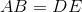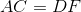, and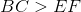, it follows that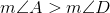, so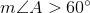.

Also, since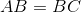, by the Isosceles Triangle Theorem,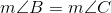. Since, and the sum of the measures of the angles of a triangle is, it follows that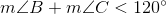Substituting and solving: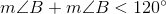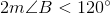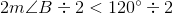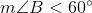.

2 Next →

### All ISEE Upper Level Quantitative Resources